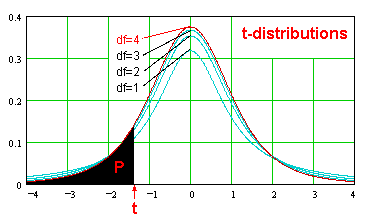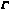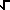Dave's Math Tables: t-distributions(Math | Stat | Distributions | t-Distributions)The t-distribution, with n degrees of freedom, is given by the equation:

f(t) = [((n + 1)/2) (1 + x^2 / n)^(-n/2 - 1/2) ] / [(n/2)(PI n) ] (See also Gamma Function.)